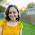## Sunday, July 8, 2012

### Calc Teachers--I need you!

My summer calculus classes are coming to a close (two weeks left--gah!).  For both finals (Business Calc and Calc I), I'm planning on having a computational part and a conceptual/theoretical/write-it-out-in-words part.   I'm working on questions for the latter part, and I need your help.  What other major (mostly differential) calculus concepts would you add?  Are any of the questions unclear?  All help is greatly appreciated!

PS:  These questions are not written in any specific order.

1. Describe what it means for a function to be continuous at a point x=c.  There should be something about a limit in your answer.
2. A function f attains both an absolute minimum and an absolute maximum on an interval as long as two conditions are met, as described by the Extreme Value Theorem.  (1) Name the two conditions.  (2) Sketch a situation in which the conditions are not met and in which the function does not attain either an absolute minimum or maximum (or both).
3. A function can attain absolute extrema at endpoints and at critical values.  What's a critical value?
4. For a function to be differentiable at a point, it must be _______________ and _____________ at that point.  Give an example of a function (in function notation, not a sketch) that is not differentiable at at least one point.  State that point.
5. Which is true:  For a function to be differentiable, it also must be continuous; For a function to be continuous, it also must be differentiable.
6. Which is true:  For a function to be integrable, it also must be continuous; For a function to be continuous, it also must be integrable.
7. If f '  is positive over an open interval, what can you tell me about f on this interval?
8. If f '' is negative over an open interval, what can you tell me about f on this interval?
9. In your own words, describe what a derivative is and what it can be used for.
10. In your own words, describe what an integral is and what it can be used for.
11. What two words did we say summarize differential calculus?
12. In words, the Mean Value Theorem says that if we look at an interval [a,b], then there is at least one point in the interval (call it c) such that the __________________ rate of change between a and b is equal to the __________________ rate of change at c.
13. Name the two inventors of calculus.  Which one do you like better?
14. Describe what each of the three parts of the following statement mean as they relate to area:
f(x)dx

1.Indefinite integrals refer to anti-derivatives, not area. If your symbol had an a and b at the top and bottom of the integral sign, then you could ask what each of the 5 parts means, and that's a great question to ask.

I like questions 7-10 and 14 best. I want to have more questions like these on my tests.

2.Yes, you're absolutely right about #14. Thankfully I noticed that before I gave out the review! I still wrote "3 parts," (the entire definite integral being "1 part") but breaking it into five may be even better.

I found a couple other great questions on an AP Calc study guide. I'll post those soon!

1.Posted! http://www.epsilon-delta.org/2012/08/i-heart-these-two-calc-problems.html

Tell me what you think!